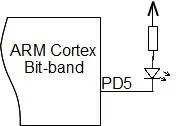# Bit Band operations with ARM Cortex microcontrollers

I got few questions from our readers about the bit-band feature in ARM Cortex microcontrollers. It may seem to be a prominent topic, still may lead to come confusion while using bit-banding. So let’s look at this feature a little bit closer.## Why use bit band

Simply speaking Bit banding method allows performing atomic bitwise operations to memory areas. Why use bit banding? The most straightforward answer is because ARM Cortex doesn’t have something like BIT CSET or BIT CLEAR commands like most of the 8-bit microcontrollers do. So this is somewhat a workaround solution. Another question may rise – Why not using the read-modify-write method? Again this method is not reliable in some cases. For instance f there is an interrupt during this operation it can cause data corruption. Other situation may occur in embedded OS when different tasks may modify the same memory location. So we want a method that allows setting or clear individual bits with a single instruction. This is where the bit band method helps.

If you open any ARM Cortex documentation or datasheet, you will find that bit-band can be performed in two memory regions – the first 1MB SRAM region (from address 20000000h to 200FFFFFh) and first 1MB peripheral region (from address 40000000h to 400FFFFFh). What does this means? This means that bitwise operations can be performed to RAM and peripherals like ports and other peripheral registers.

## How bit band method works

Bit band mechanism works by using a separate memory region called bit-band alias. Alias regions are located far from available RAM or actual peripherals.As you can see for RAM, this region starts at address 22000000h which is from 31MB. This is a safe location as ARM internal SRAM will not likely reach 32MB. The same situation is with peripheral region. It also starts are a 31MB location (at address 42000000h).

Using bit-band alias memory region we can access individual bits of RAM or peripherals. Each bit in memory is addressed using 32-bit bit-band address in alias memory region.How to calculate alias address to any bit in RAM location? For this, you can do a simple calculation. Let us say we want to access peripheral bits. We know that peripheral area starts at address 40000000h. So we call this address as Peripheral Base; then peripheral Alias Base is 42000000h. Let’s say we want to write 1 to PORT D 5th pin 1. Then we need to set this bit to 1; From memory map, we find that PORT D output register address is 4001140Ch. So this is 1140Ch – th byte in peripheral memory. Since each byte in memory offsets address by 20h in alias memory region, we can calculate that PORTD output register alias starts at 1140Ch * 20h. Then we need to add a 5th bit: + 5×4. So our alias address for PORT D output register GPIOD_ODR pin 5 is:

42000000h + 1140Ch * 20h + 5 * 4 = 42228194h

In C program we can define this bit as a pointer and use it to set or clear this bit:

`#define PortDBit5 (*((volatile unsigned long *) 0x42228194))`

Then anywhere in the program when you need to set this bit to 1 you can write:

`PortDBit5 = 1;`

Or write zero:

`PortDBit5 = 0;`

In C program you can prepare a MACRO to calculate the bit-band location for particular bit location.

```#define PERIPHERAL_BASE 0x40000000
#define BITBAND_PERIPHERAL_BASE 0x42000000
#define BITBAND_PERIPHERAL(a,b) (BITBAND_PERIPHERAL_BASE + (a-PERIPHERAL_BASE)*0x20+(b*4))
#define GPIOD_ODR 0x4001140C
//create a pointer to bit 5 in bit band location
#define PORTDBIT5 (*((volatile unsigned long *)(BITBAND_PERIPHERAL(GPIOD_ODR,5))))
//use it somewhere to set bit to 1
int main()
{
//...
PORTDBIT5 = 1;
}```

Now you see how it’ simple. If you used to read modify write operation, you would risk getting data corruption in some cases. Also, read-modify-write takes about twice as long as using bit band based set and clear. If you need to set multiple bits at the time, then you lose performance with bit band operation. So if there is no risk of data corruption (like interrupts won’t tend to change the same data), then read-modify-write is better to use when multiple bits are changed. Wise use of this method can boost some performance on a more significant scale.

Also, it is worth to mention that bit band operations can be performed for half word and byte sized memory locations. Then the difference is in the alias address algorithm. Instead, you would use multiplier 2 for half word and 1 for byte.

This site uses Akismet to reduce spam. Learn how your comment data is processed.